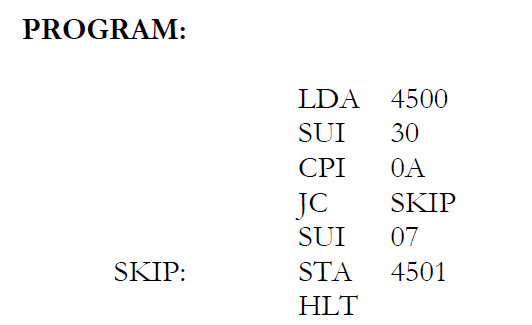# ASCII to HEX Conversion program in 8085 – PhysicsTeacher

In this post, we will see how to convert a given ASCII Character into its equivalent Hexa Decimal (HEX) number using the 8085 microprocessor program or instruction set.

## Algorithm for ASCII to HEX Conversion with 8085 microprocessor

1. Load the given data in A register
2. Subtract 30 H from A register
3. Compare the content of A register with 0A H
4. If A < 0A H, jump to step6. Else proceed to next step.
5. Subtract 07 H from A register
6. Store the result
7. Terminate the program

## 8085 Program to convert ASCII to Hexa Decimal number | ASCII to HEX program code in 8085

LDA 4500
SUI 30
CPI 0A
JC SKIP
SUI 07
SKIP: STA 4501
HLTProgram to convert ASCII Character into its equivalent Hexa Decimal number using the 8085 microprocessor (ASCII to HEX Conversion)

Input: 4500 31
Output: 4501 0B

## Result

Thus the given ASCII character was converted into its equivalent Hexa Value using the 8085 microprocessor program.

See also  Square of a number using a look-up table (assembly language program)
Scroll to top
error: physicsTeacher.in